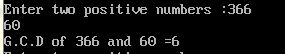UncleCoder.com

UncleCoder.com

Free programming examples and instructions

# C Program to find GCD of two numbers

## C Program for how to find GCD of two numbers

by Krishna

Posted on 27 Jul 2018 Category: C Views: 971

C Program to find GCD of two numbers

The  GCD of two integers is the largest integer that can exactly divide both numbers (without a remainder). Below shows the program to find the GCD of two numbers.

``````#include <stdio.h>
int main()
{
int n1, n2, i, gcd;
printf("Enter two positive numbers: ");
scanf("%d %d", &n1, &n2);
for(i=1; i <= n1 && i <= n2; ++i)
{
// Checks if i is factor of both integers
if(n1%i==0 && n2%i==0)
gcd = i;
}
printf("G.C.D of %d and %d is %d", n1, n2, gcd);
return 0;
}``````

In this program, two integers entered by the user are stored in variable n1 and n2.Then, `for` loop is iterated until i is less than n1 and n2. In each iteration, if both n1 and n2 are exactly divisible by i, the value of i is assigned to gcd. When the `for` loop is completed, the greatest common divisor of two numbers is stored in variable gcd. The output is also shown below.

OUTPUT